# 串口通讯调试与开发

## 串口模拟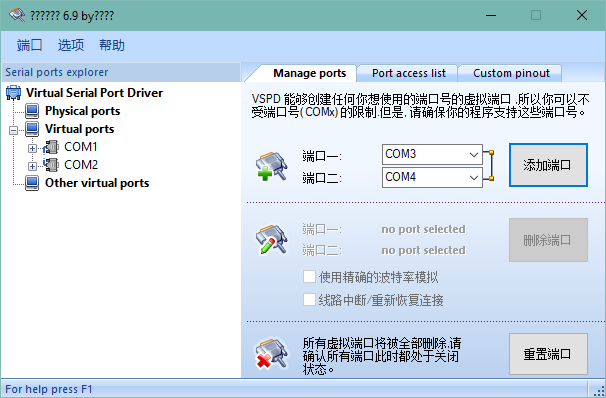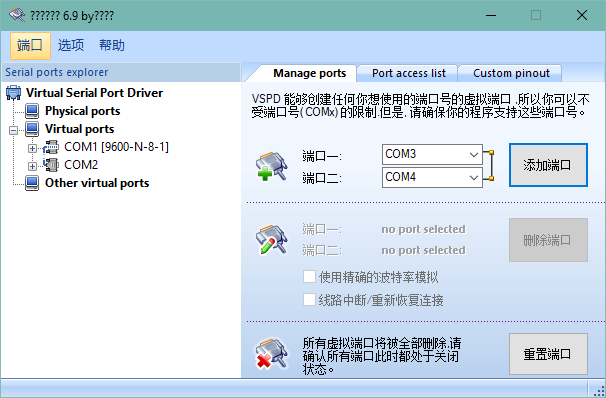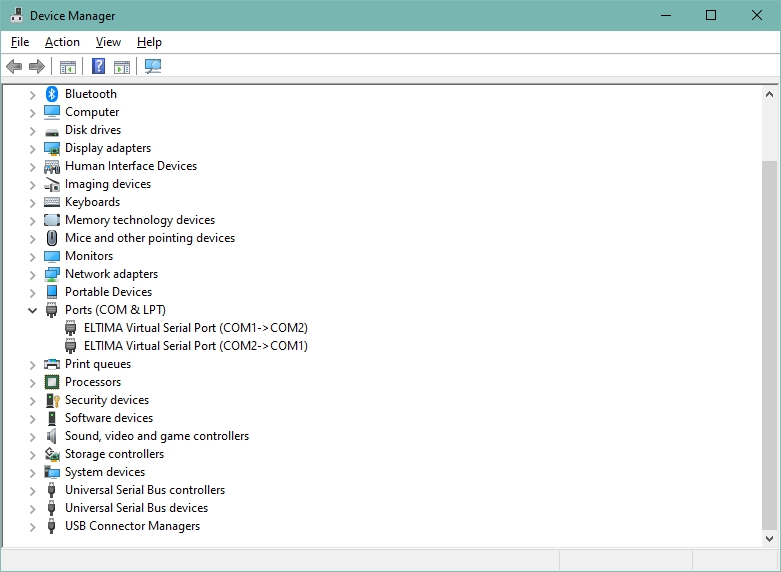## 串口调试工具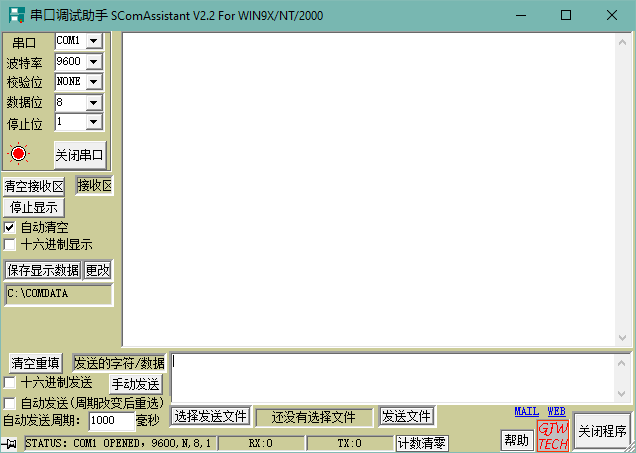1. 偶尔会发生崩溃，这是串口调试工具的问题；
2. 只能使用COM1-COM4这几个串口，有局限性；
3. 与某些需要检测串口监听状态的仪器连接，虽然已经打开串口但是可能仪器仍然提示连接失败；

## 串口监视精灵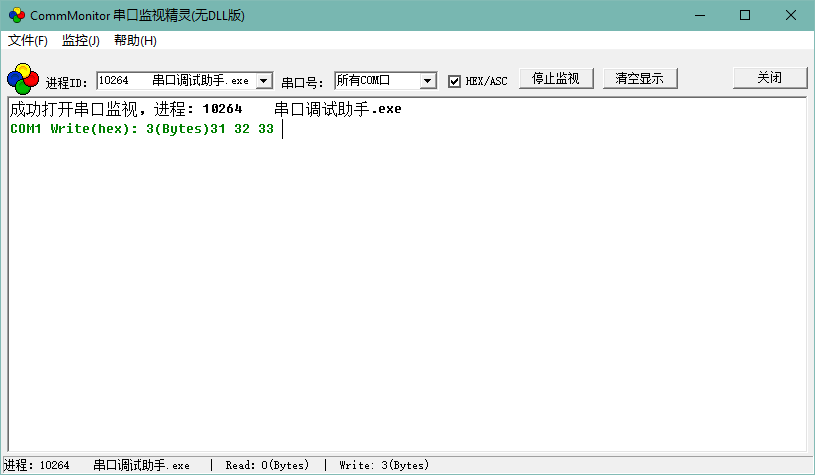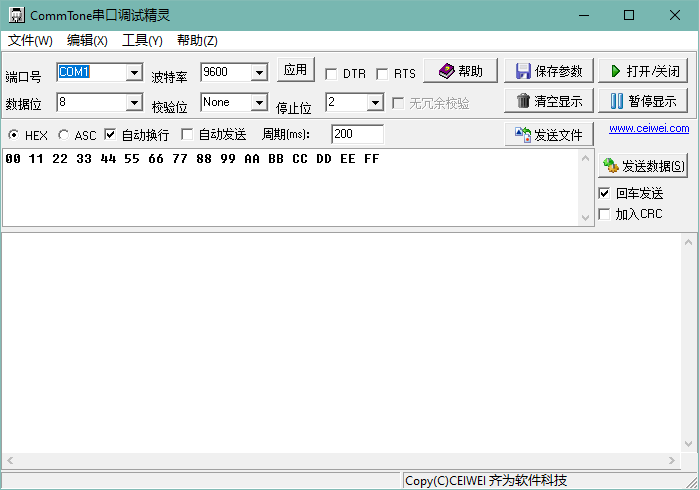## 串口通讯开发

### 获取当前电脑的串口

using System;
using System.IO.Ports;

namespace SerialPortTestConsole
{
class Program
{
static void Main(string[] args)
{
string[] ports = SerialPort.GetPortNames();
foreach (string port in ports)
{
Console.WriteLine(port);
}
}
}
}


### 串口数据收发

using System;
using System.IO.Ports;
using System.Text;

namespace SerialPortTestConsole
{
class Program
{
static void Main(string[] args)
{
//注意：以下为串口初始化常用的属性，赋值为默认值，如果没有赋值属性默认也是这些值
SerialPort port = new SerialPort()
{
PortName = "COM1",//端口号
BaudRate = 9600,//波特率
DataBits = 8,//数据位
Parity = Parity.None,//校验位
StopBits = StopBits.One,//停止位
Encoding = Encoding.ASCII,//文本转换编码
WriteBufferSize = 2048,//输出缓存
WriteTimeout = -1,//写超时
RtsEnable = false,//RTS信号：建议启用
DtrEnable = false,//DTR信号：建议启用
};

//数据接收事件 输出到控制台
{
{
byte[] buffer = new byte[length];
Console.WriteLine(port.Encoding.GetString(buffer));
}
});

//打开串口
if (!port.IsOpen)
{
try
{
port.Open();
Console.WriteLine($"串口打开成功：{port.PortName}"); } catch (Exception exception) { Console.WriteLine($"打开串口失败：{exception.Message}");
}
}

if (port.IsOpen)
{
//输入串口发送内容
string input = "";
do
{
Console.WriteLine("请输入要发送的内容：");
if (!string.IsNullOrEmpty(input))
{
byte[] buffer = port.Encoding.GetBytes(input);
port.Write(buffer, 0, buffer.Length);
}
} while (!string.IsNullOrEmpty(input));

//关闭串口释放资源
port.Close();
}
port.Dispose();
}
}
}


### 一个简单的调试工具

SerialPort的属性/方法/事件基本没有什么好说的了，这里主要需要了解HEX数据的转换：

/// <summary>
/// 字符串拓展方法
/// </summary>
public static partial class StringExtension
{
/// <summary>
/// 将指定字符串转换为十六进制Hex字符串形式。
/// </summary>
/// <param name="input">要转换的原始字符串。</param>
/// <param name="e">编码</param>
/// <returns>转换后的内容</returns>
public static string ToHex(this string input, Encoding e = null)
{
e = e ?? Encoding.UTF8;
byte[] byteArray = e.GetBytes(input);
return BitConverter.ToString(byteArray).Replace('-', ' ');
}

/// <summary>
/// 将十六进制Hex字符串转换为原始字符串。
/// </summary>
/// <param name="input">十六进制字符串内容</param>
/// <param name="e">编码</param>
/// <returns>原始字符串内容</returns>
public static string ReHex(this string input, Encoding e = null)
{
e = e ?? Encoding.UTF8;
input = Regex.Replace(input, "[^0-9A-F]", "");
if (input.Length <= 0) return "";
byte[] vBytes = new byte[input.Length / 2];
for (int i = 0; i < vBytes.Length; i++)
vBytes[i] = byte.Parse(input.Substring(i * 2, 2), NumberStyles.HexNumber);
return e.GetString(vBytes);
}

/// <summary>
/// 将十六进制Hex字符串转换为二进制数据。
/// </summary>
/// <param name="input">十六进制字符串内容</param>
/// <returns>原始字符串内容</returns>
public static byte[] ReHexToBuffer(this string input)
{
input = Regex.Replace(input, "[^0-9A-F]", "");
if (string.IsNullOrEmpty(input)) return new byte;
byte[] vBytes = new byte[input.Length / 2];
for (int i = 0; i < vBytes.Length; i++)
vBytes[i] = byte.Parse(input.Substring(i * 2, 2), NumberStyles.HexNumber);
return vBytes;
}
}

/// <summary>
/// 二进制数据拓展方法
/// </summary>
public static partial class BufferExtension
{
/// <summary>
/// 将指定二进制数据转换为十六进制Hex字符串形式。
/// </summary>
/// <param name="input">要转换的二进制数据。</param>
/// <returns>转换后的内容</returns>
public static string ToHex(this byte[] input)
{
return BitConverter.ToString(input).Replace('-', ' ');
}
}